## FCSys.Regions

3D arrays of discrete, interconnected subregions

### Information

Extends from Modelica.Icons.Package (Icon for standard packages).

### Package Content

NameDescription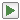Examples Examples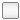AnFPs Anode flow platesAnGDLs Anode gas diffusion layersAnCLs Anode catalyst layersPEMs Proton exchange membranesCaCLs Cathode catalyst layersCaGDLs Cathode gas diffusion layersCaFPs Cathode flow plates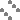Region Base model for a 3D array of subregions

##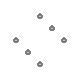FCSys.Regions.Region

Base model for a 3D array of subregions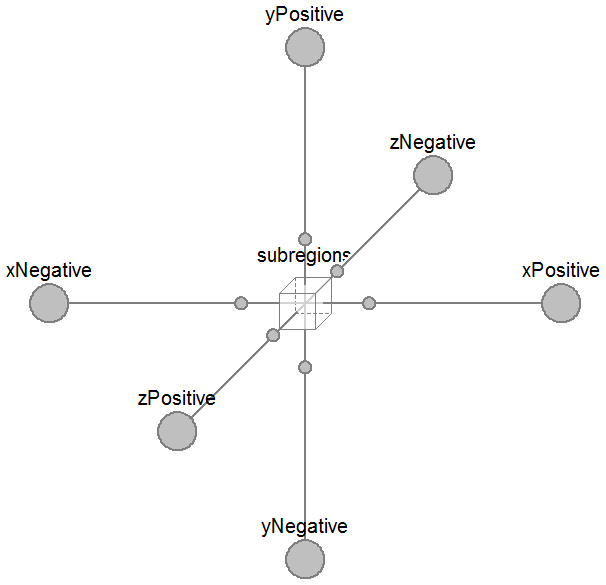### Information

If Lx is an empty vector (e.g., `zeros(0)`, `ones(0)`, or `fill(1, 0)`), then there are no subregions along the x axis and the boundaries along the x axis are directly connected. The same applies to the other axes.

### Parameters

TypeNameDefaultDescription
replaceable model SubregionSubregions.SubregionBase subregion model
Geometry
LengthL_x[:]{U.cm}Lengths along the x axis [L]
LengthL_y[:]{U.cm}Lengths along the y axis [L]
LengthL_z[:]{U.cm}Lengths across the z axis [L]
Assumptions
Included transport axes
BooleaninclTransXtrueX
BooleaninclTransYtrueY
BooleaninclTransZtrueZ

### Connectors

TypeNameDescription
replaceable model SubregionBase subregion model
BoundaryBusxNegative[n_y, n_z]Negative boundary along the x axis
BoundaryBusxPositive[n_y, n_z]Positive boundary along the x axis
BoundaryBusyNegative[n_x, n_z]Negative boundary along the y axis
BoundaryBusyPositive[n_x, n_z]Positive boundary along the y axis
BoundaryBuszNegative[n_x, n_y]Negative boundary along the z axis
BoundaryBuszPositive[n_x, n_y]Positive boundary along the z axis

### Modelica definition

```model Region "Base model for a 3D array of subregions"
import FCSys.Utilities.Coordinates.cartWrap;
// extends FCSys.Icons.Names.Top3;
// extends FCSys.Icons.Names.Top6;

// Geometric parameters
parameter Q.Length L_x[:]={U.cm} "Lengths along the x axis";
parameter Q.Length L_y[:]={U.cm} "Lengths along the y axis";
parameter Q.Length L_z[:]={U.cm} "Lengths across the z axis";

final parameter Integer n_x=size(L_x, 1)
"Number of sets of subregions along the x axis";
final parameter Integer n_y=size(L_y, 1)
"Number of sets of subregions along the y axis";
final parameter Integer n_z=size(L_z, 1)
"Number of sets of subregions along the z axis";

// Assumptions
// -----------
// Included transport axes
parameter Boolean inclTransX=true "X";
parameter Boolean inclTransY=true "Y";
parameter Boolean inclTransZ=true "Z";

// Auxiliary parameters (for analysis only)
final parameter Q.Length L[Axis]={sum(L_x),sum(L_y),sum(L_z)} if
hasSubregions "Length";
final parameter Q.Area A[Axis]={L[cartWrap(axis + 1)]*L[cartWrap(axis + 2)]
for axis in Axis} if hasSubregions "Cross-sectional areas";
final parameter Q.Volume V=product(L) if hasSubregions "Volume";

replaceable model Subregion = Subregions.Subregion constrainedby
FCSys.Subregions.PartialSubregion(
final inclTransX=inclTransX,
final inclTransY=inclTransY,
final inclTransZ=inclTransZ) "Base subregion model";
// Note:  In Dymola 2014, the inclTransX, inclTransY, and inclTransZ parameters
// still appear in the parameter dialog even though they are final.

Subregion subregions[n_x, n_y, n_z](final L={{L_x[i_x],L_y[i_y],L_z[i_z]}
for i_z in 1:n_z, i_y in 1:n_y, i_x in 1:n_x}) if hasSubregions
"Instances of the subregion model";
Connectors.BoundaryBus xNegative[n_y, n_z] if inclTransX
"Negative boundary along the x axis";
Connectors.BoundaryBus xPositive[n_y, n_z] if inclTransX
"Positive boundary along the x axis";
Connectors.BoundaryBus yNegative[n_x, n_z] if inclTransY
"Negative boundary along the y axis";
Connectors.BoundaryBus yPositive[n_x, n_z] if inclTransY
"Positive boundary along the y axis";
Connectors.BoundaryBus zNegative[n_x, n_y] if inclTransZ
"Negative boundary along the z axis";
Connectors.BoundaryBus zPositive[n_x, n_y] if inclTransZ
"Positive boundary along the z axis";

protected
final parameter Boolean hasSubregions=n_x > 0 and n_y > 0 and n_z > 0
"true, if there are any subregions";

equation
// X axis
connect(xNegative, subregions[1, :, :].xNegative);
if inclTransX then
for i in 1:n_x - 1 loop
connect(subregions[i, :, :].xPositive, subregions[i + 1, :, :].xNegative)
"Connection b/w neighboring subregions (not shown in the diagram)";
end for;
end if;
connect(subregions[n_x, :, :].xPositive, xPositive);
if n_x == 0 then
connect(xNegative, xPositive)
"Direct pass-through (not shown in the diagram)";
end if;

// Y axis
connect(yNegative, subregions[:, 1, :].yNegative);
if inclTransY then
for i in 1:n_y - 1 loop
connect(subregions[:, i, :].yPositive, subregions[:, i + 1, :].yNegative)
"Connection b/w neighboring subregions (not shown in the diagram)";
end for;
end if;
connect(subregions[:, n_y, :].yPositive, yPositive);

if n_y == 0 then
connect(yNegative, yPositive)
"Direct pass-through (not shown in the diagram)";
end if;

// Z axis
connect(zNegative, subregions[:, :, 1].zNegative);
if inclTransZ then
for i in 1:n_z - 1 loop
connect(subregions[:, :, i].zPositive, subregions[:, :, i + 1].zNegative)
"Connection b/w neighboring subregions (not shown in the diagram)";
end for;
end if;
connect(zPositive, subregions[:, :, n_z].zPositive);
if n_z == 0 then
connect(zNegative, zPositive)
"Direct pass-through (not shown in the diagram)";
end if;
// TODO:  Once primitivesVisible is supported by Modelica tools (not
// supported as of Dymola 2014), complete the icon of this model.
// Until then, the icon should be blank so that the layer models (AnFP, AnGDL, etc.)
// are not affected.
end Region;
```

##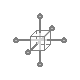FCSys.Regions.Region.Subregion

Base subregion model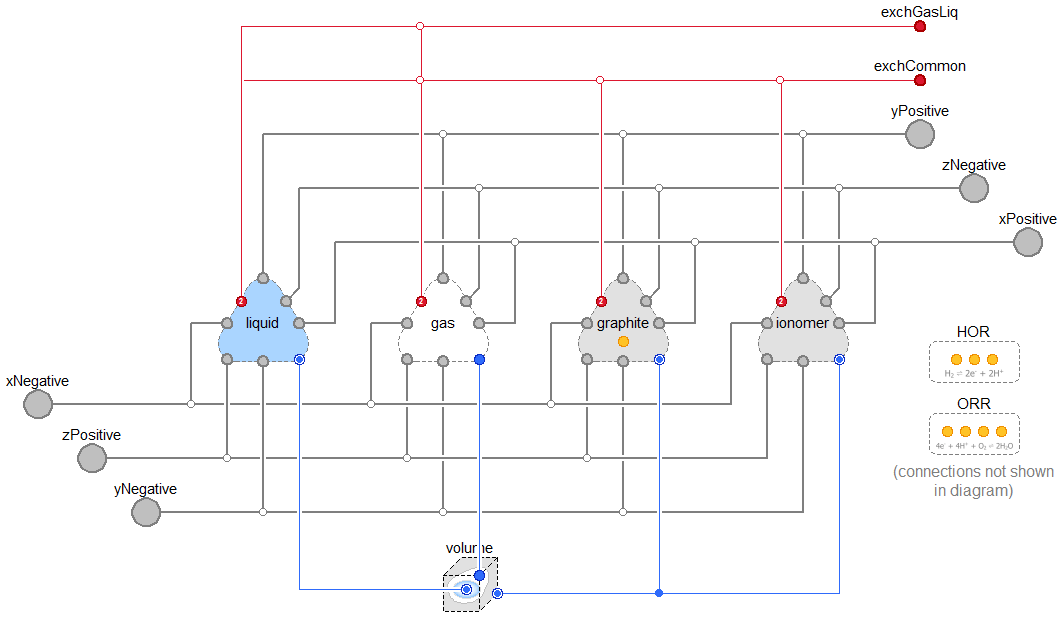### Parameters

TypeNameDefaultDescription
CapillaryVolumevolume Volume with capillary pressure included
Geometry
LengthL[Axis]{U.cm,U.cm,U.cm}Lengths [L]
Phases (click to edit)
Gasgas Gas
Graphitegraphite Graphite
Ionomerionomer Ionomer
Liquidliquid Liquid
Independence factors
ExchangeParamscommon Among all phases
ExchangeParamsgasLiq Between gas and liquid
Assumptions
Included transport axes
BooleaninclTransXtrueX
BooleaninclTransYtrueY
BooleaninclTransZtrueZ

### Connectors

TypeNameDescription
BoundaryBusxNegativeNegative boundary along the x axis
BoundaryBusyNegativeNegative boundary along the y axis
BoundaryBuszNegativeNegative boundary along the z axis
BoundaryBusxPositivePositive boundary along the x axis
BoundaryBusyPositivePositive boundary along the y axis
BoundaryBuszPositivePositive boundary along the z axis

### Modelica definition

```replaceable model Subregion = Subregions.Subregion constrainedby
FCSys.Subregions.PartialSubregion(
final inclTransX=inclTransX,
final inclTransY=inclTransY,
final inclTransZ=inclTransZ) "Base subregion model";
```